# Why India has 50 Hz Power System and US has 60 Hz 110 Volts Power System

### Why India has 50 Hz Power system and the US has 60 Hz 110 Volts power system.

India supplies 50 Hz, 230 Volts, Alternating Current for all of their citizen’s household and other domestic application, but in the US, Canada, Brazil such countries are using 60 Hz frequency, 110 Volts AC powers system, why? Let’s see the real reason behind it, and Also which system is more efficiency.

Let see the Possible reason of Why India has 50 Hz Power system:

### 1. History

What we have said in our title, that is actually wrong, because India did not choose their system to operate with the 50 Hz, because Indian standard is a color Xerox of British standard, suppose if British chose 60 Hz power system (at that time of British ruling in India) means, India definitely follows the British standard as 60 Hz but they did not, so that, instead of saying why India have 50 Hz power system, we should say why British have chosen 50 Hz Power system.

First,

### What is 50 Hz, 230 Volts AC?

It means the potential difference (voltage) between two terminals is 230 Volts and 50Hz means the AC sinusoidal waveform reaches 50 cycles per second. Which means the sine wave changes its direction 50 times for each second.

### What is 60 Hz, 110 volts AC?

The potential difference between two terminals is 110 Volts and the frequency of the incoming supply is 60 Hz (reaches 60 cycles per second).

Note: Both the power system has advantages and disadvantages, but 50 Hz power system have some extra advantage over 60 Hz power system.

The advantage of 50 Hz Power system over 60 Hz power system?

## 1. Reducing loss

In this, we can reduce Constant loss and variable loss, let see how?

Reducing Constant loss:

As we know the constant loss is nothing but a hysteresis loss + eddy current loss. Here the eddy current loses is directly proportional to the square of generation frequency and hysteresis loss is directly proportional to the frequency, Hence, the reducing frequency of the generation reduces the total constant loses of the machine. Also, the impact will reflect on Motor’s energy consumption.

Here, Eddy current loss,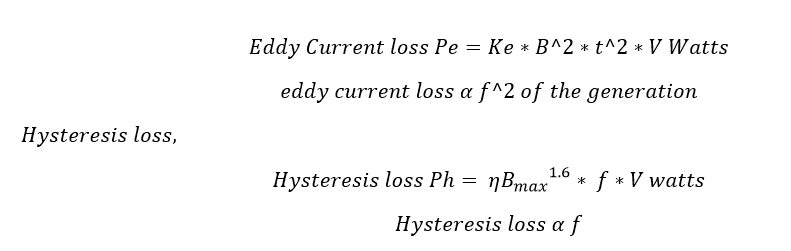### Reducing Variable loss:

In this, by reducing the frequency of the alternating current, we can reduce the impedance loss of the circuit. Let see how?

As we know Impedance Z is the vector sum of resistor R and inductive reactance Xl of the circuit.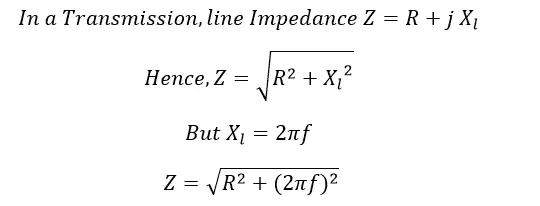From the equation Xl you can see the reducing frequency of the circuit, the inductive reactance of the circuit get reduces. Hence the Total impedance of the circuit also reduces.

From the equation the loss of the circuit also reduces.

1. Increasing the supply Voltage in the utility side decreases the current of the circuit. Hence, line loss reduces. This phenomenon can be obtained from Ohms Law.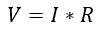Increase Voltage V, decrease the current (flow of electron or charges) flowing through the circuit. That’s why British chose to supply 240 volts for domestic supply.

### 3. Size of the conductor:

If your circuit carries the larger quantity of current, you should increase the size of the conductor. If you supply a high voltage, the total current reduces and you do not want to go for larger size conductor.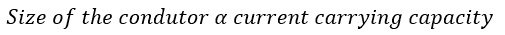Copper and aluminum are mainly used in the electrical system to carry current to the other circuit.

Note: Current in open circuit is zero and current in short circuit is high.

### 4. By reducing the frequency of the system, you can increase your power generation.

Example: Take a steam power plant which is running with coal and the coal to the steam ratio of the boiler is 4.5 Tons stream per ton of coal. But the Steam to power ratio is 2.5 Tons per MW (one Megawatt = 10,00,000 watts), hence we can generate around 1.5-1.7 MW of power from one ton of coal. The coal cost is Rs. 5600 /- Imported steaming coal, at the same time the power export cost is 1 unit per Rs. 1.25 to 5 /-, consider we export 1.6 MW for one ton of coal, the electricity output is Rs. 1600 units * 3.5 (appx. Rs 3.5 as unit cost for export). The total Value = 5600/-, Here the output is zero.

To avoid this, we have to reduce the auxiliary consumption. Auxiliary consumption can be reduced by reducing losses of the plant.

### 6. Insulation cost:

When we increase the frequency of the generation, simultaneously, the output voltage also increases. Hence the insulation strength to increase the increasing voltage.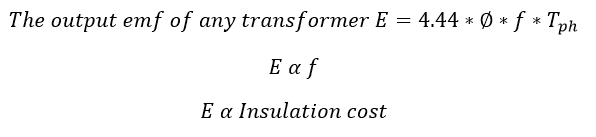### 7. The speed of the machine:

The speed of the machine increase when you increase the frequency of the system.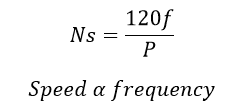Hence you have to design a machine to carry extra 20 % of the rated speed.

8. Corona Losses also decrease when the frequency of the system decreases. So that 50 Hz power system have less corona losses.

## The disadvantage of 50 Hz Power system.

• The size of the machine gets increased by decreasing the frequency, hence you have to increase the size of each machine you install in the feature.
• The 240volts, 50 Hz system have less safety against electrical shocking because high voltage system easily penetrates or create a path to current flow. But low voltage system does not contain such hazardous activity.